# Active Contours using Parameteric Curves¶

Important: Please read the installation page for details about how to install the toolboxes. $\newcommand{\dotp}{\langle #1, #2 \rangle}$ $\newcommand{\enscond}{\lbrace #1, #2 \rbrace}$ $\newcommand{\pd}{ \frac{ \partial #1}{\partial #2} }$ $\newcommand{\umin}{\underset{#1}{\min}\;}$ $\newcommand{\umax}{\underset{#1}{\max}\;}$ $\newcommand{\umin}{\underset{#1}{\min}\;}$ $\newcommand{\uargmin}{\underset{#1}{argmin}\;}$ $\newcommand{\norm}{\|#1\|}$ $\newcommand{\abs}{\left|#1\right|}$ $\newcommand{\choice}{ \left\{ \begin{array}{l} #1 \end{array} \right. }$ $\newcommand{\pa}{\left(#1\right)}$ $\newcommand{\diag}{{diag}\left( #1 \right)}$ $\newcommand{\qandq}{\quad\text{and}\quad}$ $\newcommand{\qwhereq}{\quad\text{where}\quad}$ $\newcommand{\qifq}{ \quad \text{if} \quad }$ $\newcommand{\qarrq}{ \quad \Longrightarrow \quad }$ $\newcommand{\ZZ}{\mathbb{Z}}$ $\newcommand{\CC}{\mathbb{C}}$ $\newcommand{\RR}{\mathbb{R}}$ $\newcommand{\EE}{\mathbb{E}}$ $\newcommand{\Zz}{\mathcal{Z}}$ $\newcommand{\Ww}{\mathcal{W}}$ $\newcommand{\Vv}{\mathcal{V}}$ $\newcommand{\Nn}{\mathcal{N}}$ $\newcommand{\NN}{\mathcal{N}}$ $\newcommand{\Hh}{\mathcal{H}}$ $\newcommand{\Bb}{\mathcal{B}}$ $\newcommand{\Ee}{\mathcal{E}}$ $\newcommand{\Cc}{\mathcal{C}}$ $\newcommand{\Gg}{\mathcal{G}}$ $\newcommand{\Ss}{\mathcal{S}}$ $\newcommand{\Pp}{\mathcal{P}}$ $\newcommand{\Ff}{\mathcal{F}}$ $\newcommand{\Xx}{\mathcal{X}}$ $\newcommand{\Mm}{\mathcal{M}}$ $\newcommand{\Ii}{\mathcal{I}}$ $\newcommand{\Dd}{\mathcal{D}}$ $\newcommand{\Ll}{\mathcal{L}}$ $\newcommand{\Tt}{\mathcal{T}}$ $\newcommand{\si}{\sigma}$ $\newcommand{\al}{\alpha}$ $\newcommand{\la}{\lambda}$ $\newcommand{\ga}{\gamma}$ $\newcommand{\Ga}{\Gamma}$ $\newcommand{\La}{\Lambda}$ $\newcommand{\si}{\sigma}$ $\newcommand{\Si}{\Sigma}$ $\newcommand{\be}{\beta}$ $\newcommand{\de}{\delta}$ $\newcommand{\De}{\Delta}$ $\newcommand{\phi}{\varphi}$ $\newcommand{\th}{\theta}$ $\newcommand{\om}{\omega}$ $\newcommand{\Om}{\Omega}$

This tour explores image segmentation using parametric active contours.

In :
addpath('toolbox_signal')


## Parameteric Curves¶

In this tours, the active contours are represented using parametric curve $\ga : [0,1] \rightarrow \RR^2$.

This curve is discretized using a piewise linear curve with $p$ segments, and is stored as a complex vector of points in the plane $\ga \in \CC^p$.

Initial polygon.

In :
gamma0 = [0.78 0.14 0.42 0.18 0.32 0.16 0.75 0.83 0.57 0.68 0.46 0.40 0.72 0.79 0.91 0.90]' + ...
1i* [0.87 0.82 0.75 0.63 0.34 0.17 0.08 0.46 0.50 0.25 0.27 0.57 0.73 0.57 0.75 0.79]';


Number of points of the discrete curve.

In :
p = 256;


Shortcut to re-sample a curve according to arc length.

In :
curvabs = @(gamma)[0;cumsum( 1e-5 + abs(gamma(1:end-1)-gamma(2:end)) )];
resample1 = @(gamma,d)interp1(d/d(end),gamma,(0:p-1)'/p, 'linear');
resample = @(gamma)resample1( [gamma;gamma(1)], curvabs( [gamma;gamma(1)] ) );


Initial curve $\ga_1(t)$.

In :
gamma1 = resample(gamma0);


Display the initial curve.

In :
clf;
h = plot(gamma1([1:end 1]), 'k');
set(h, 'LineWidth', 2); axis('tight'); axis('off');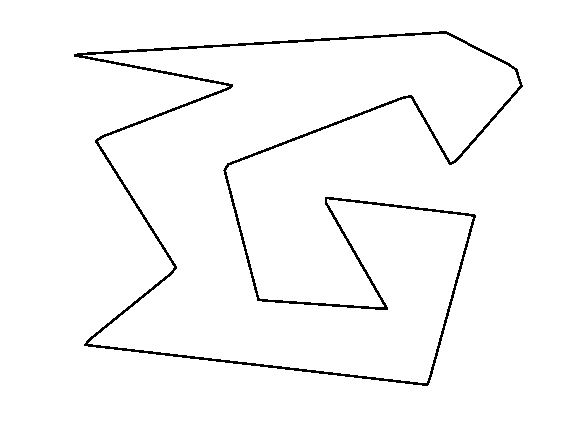Shortcut for forward and backward finite differences.

In :
BwdDiff = @(c)c - c([end 1:end-1]);
FwdDiff = @(c)c([2:end 1]) - c;
dotp = @(c1,c2)real(c1.*conj(c2));


The tangent to the curve is computed as $$t_\ga(s) = \frac{\ga'(t)}{\norm{\ga'(t)}}$$ and the normal is $n_\ga(t) = t_\ga(t)^\bot.$

Shortcut to compute the tangent and the normal to a curve.

In :
normalize = @(v)v./max(abs(v),eps);
tangent = @(gamma)normalize( FwdDiff(gamma) );
normal = @(gamma)-1i*tangent(gamma);


Move the curve in the normal direction, by computing $\ga_1(t) \pm \delta n_{\ga_1}(t)$.

In :
delta = .03;
gamma2 = gamma1 + delta * normal(gamma1);
gamma3 = gamma1 - delta * normal(gamma1);


Display the curves.

In :
clf;
hold on;
h = plot(gamma1([1:end 1]), 'k'); set(h, 'LineWidth', 2);
h = plot(gamma2([1:end 1]), 'r--'); set(h, 'LineWidth', 2);
h = plot(gamma3([1:end 1]), 'b--'); set(h, 'LineWidth', 2);
axis('tight'); axis('off');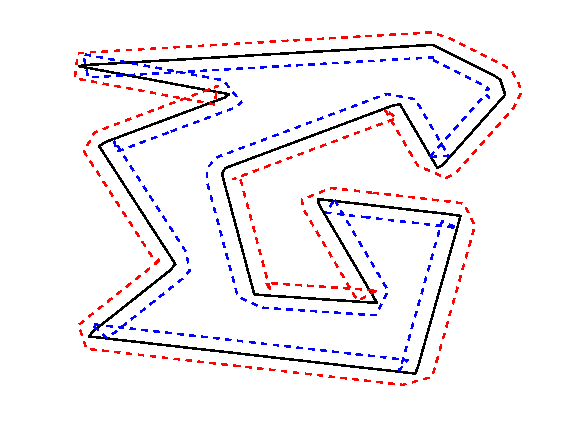## Evolution by Mean Curvature¶

A curve evolution is a series of curves $s \mapsto \ga_s$ indexed by an evolution parameter $s \geq 0$. The intial curve $\ga_0$ for $s=0$ is evolved, usually by minizing some energy $E(\ga)$ in a gradient descent $$\frac{\partial \ga_s}{\partial s} = \nabla E(\ga_s).$$

Note that the gradient of an energy is defined with respect to the curve-dependent inner product $$\dotp{a}{b} = \int_0^1 \dotp{a(t)}{b(t)} \norm{\ga'(t)} d t.$$ The set of curves can thus be thought as being a Riemannian surface.

The simplest evolution is the mean curvature evolution. It corresponds to minimization of the curve length $$E(\ga) = \int_0^1 \norm{\ga'(t)} d t$$

The gradient of the length is $$\nabla E(\ga)(t) = -\kappa_\ga(t) n_\ga(t)$$ where $\kappa_\ga$ is the curvature, defined as $$\kappa_\ga(t) = \frac{1}{\norm{\ga'(t)}} \dotp{ t_\ga'(t) }{ n_\ga(t) } .$$

Shortcut for normal times curvature $\kappa_\ga(t) n_\ga(t)$.

In :
normalC = @(gamma)BwdDiff(tangent(gamma)) ./ abs( FwdDiff(gamma) );


Time step for the evolution. It should be very small because we use an explicit time stepping and the curve has strong curvature.

In :
dt = 0.001 / 100;


Number of iterations.

In :
Tmax = 3 / 100;
niter = round(Tmax/dt);


Initialize the curve for $s=0$.

In :
gamma = gamma1;


Evolution of the curve.

In :
gamma = gamma + dt * normalC(gamma);


To stabilize the evolution, it is important to re-sample the curve so that it is unit-speed parametrized. You do not need to do it every time step though (to speed up).

In :
gamma = resample(gamma);


Exercise 1

Perform the curve evolution. You need to resample it a few times.

In :
exo1()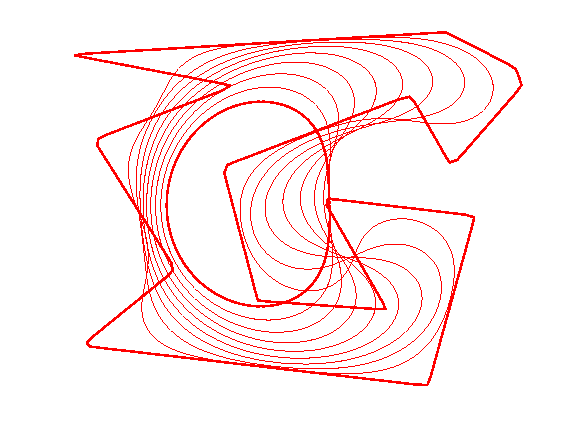In :
%% Insert your code here.


## Geodesic Active Contours¶

Geodesic active contours minimize a weighted length $$E(\ga) = \int_0^1 W(\ga(t)) \norm{\ga'(t)} d t,$$ where $W(x)>0$ is the geodesic metric, that should be small in areas where the image should be segmented.

Create a synthetic weight $W(x)$.

In :
n = 200;
nbumps = 40;
theta = rand(nbumps,1)*2*pi;
r = .6*n/2; a = [.62*n .6*n];
x = round( a(1) + r*cos(theta) );
y = round( a(2) + r*sin(theta) );
W = zeros(n); W( x + (y-1)*n ) = 1;
W = perform_blurring(W,10);
W = rescale( -min(W,.05), .3,1);


Display the metric.

In :
clf;
imageplot(W);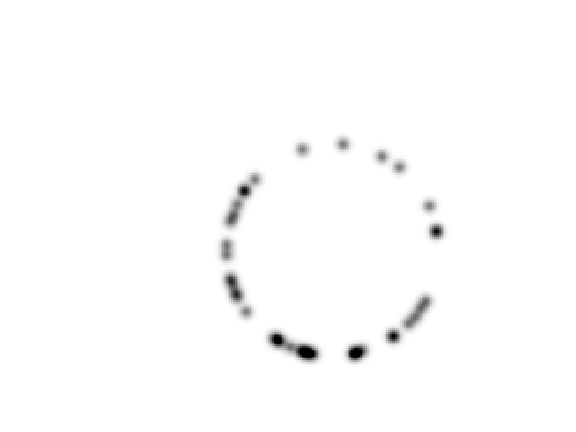Pre-compute the gradient $\nabla W(x)$ of the metric.

In :
options.order = 2;
G = G(:,:,1) + 1i*G(:,:,2);


Shortcut to evaluate the gradient and the potential along a curve.

In :
EvalG = @(gamma)interp2(1:n,1:n, G, imag(gamma), real(gamma));
EvalW = @(gamma)interp2(1:n,1:n, W, imag(gamma), real(gamma));


Create a circular curve $\ga_0$.

In :
r = .98*n/2;
p = 128; % number of points on the curve
theta = linspace(0,2*pi,p+1)'; theta(end) = [];
gamma0 = n/2*(1+1i) +  r*(cos(theta) + 1i*sin(theta));


Initialize the curve at time $t=0$ with a circle.

In :
gamma = gamma0;


For this experiment, the time step should be larger, because the curve is in $[1,n] \times [1,n]$.

In :
dt = 1;


Number of iterations.

In :
Tmax = 5000;
niter = round(Tmax/dt);


Display the curve on the back ground;

In :
lw = 2;
clf; hold on;
imageplot(W);
h = plot(imag(gamma([1:end 1])),real(gamma([1:end 1])), 'r');
set(h, 'LineWidth', lw);
axis('ij');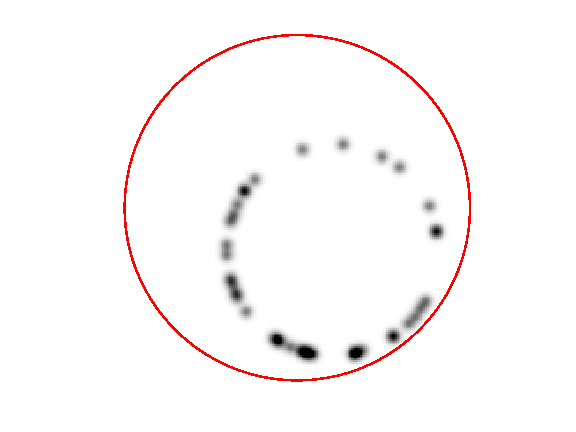The gradient of the energy is $$\nabla E(\ga) = -W(\ga(t)) \kappa_\ga(t) n_\ga(t) + \dotp{\nabla W(\ga(t))}{ n_\ga(t) } n_\ga(t).$$

Evolution of the curve according to this gradient.

In :
N = normal(gamma);
g = - EvalW(gamma).*normalC(gamma) + dotp(EvalG(gamma), N) .* N;
gamma = gamma - dt*g;


To avoid the curve from being poorly sampled, it is important to re-sample it evenly.

In :
gamma = resample( gamma );


Exercise 2

Perform the curve evolution.

In :
exo2()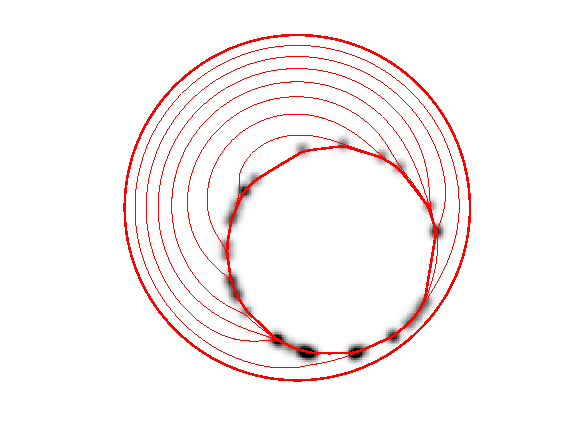In :
%% Insert your code here.


## Medical Image Segmentation¶

One can use a gradient-based metric to perform edge detection in medical images.

Load an image $f$.

In :
n = 256;
f = rescale( sum(load_image('cortex', n), 3 ) );


Display.

In :
clf;
imageplot(f);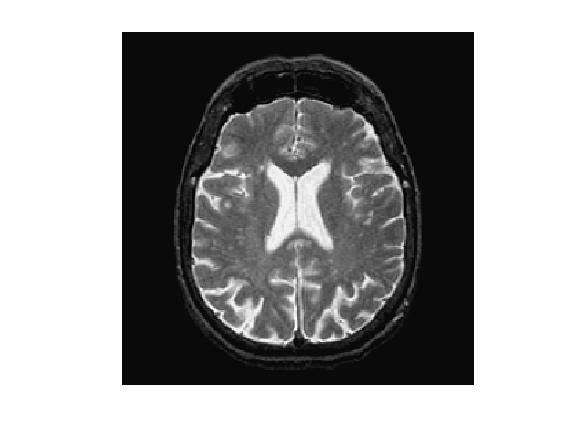An edge detector metric can be defined as a decreasing function of the gradient magnitude. $$W(x) = \psi( d \star h_a(x) ) \qwhereq d(x) = \norm{\nabla f(x)}.$$ where $h_a$ is a blurring kernel of width $a>0$.

Compute the magnitude of the gradient.

In :
options.order = 2;
d = sqrt(sum(G.^2,3));


Blur it by $h_a$.

In :
a = 3;
d = perform_blurring(d,a);


Compute a decreasing function of the gradient to define $W$.

In :
d = min(d,.4);
W = rescale(-d,.8,1);


Display it.

In :
clf;
imageplot(W);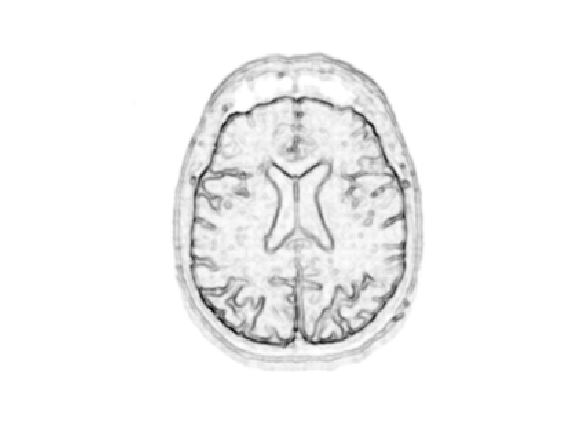Number of points.

In :
p = 128;


Exercise 3

Create an initial circle $\gamma_0$ of $p$ points.

In :
exo3()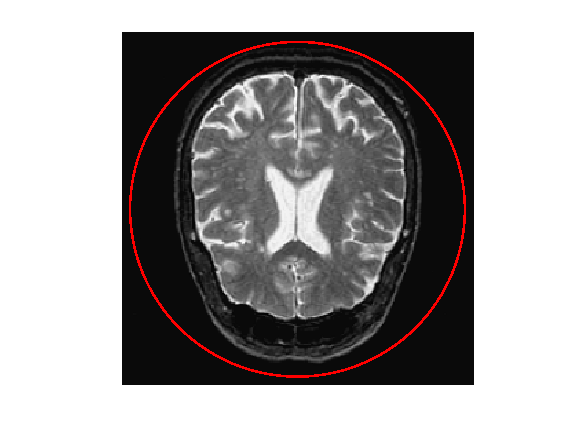In :
%% Insert your code here.


Step size.

In :
dt = 2;


Number of iterations.

In :
Tmax = 9000;
niter = round(Tmax/dt);


Exercise 4

Perform the curve evolution.

In :
exo4()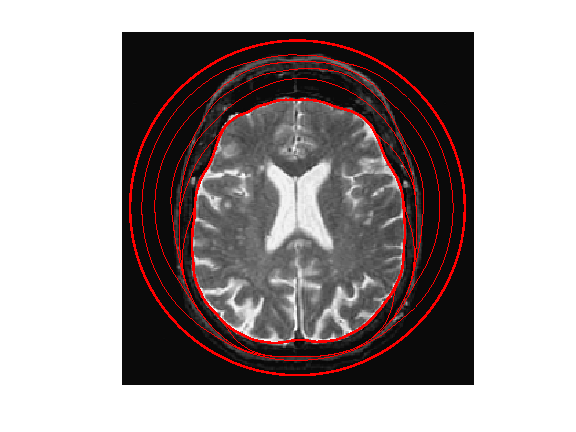In :
%% Insert your code here.


## Evolution of a Non-closed Curve¶

It is possible to perform the evolution of a non-closed curve by adding boundary constraint $$\ga(0)=x_0 \qandq \ga(1)=x_1.$$

In this case, the algorithm find a local minimizer of the geodesic distance between the two points.

Note that a much more efficient way to solve this problem is to use the Fast Marching algorithm to find the global minimizer of the geodesic length.

Load an image $f$.

In :
n = 256;
f = rescale( sum(load_image('cortex', n), 3 ) );
f = f(46:105,61:120);
n = size(f,1);


Display.

In :
clf;
imageplot(f);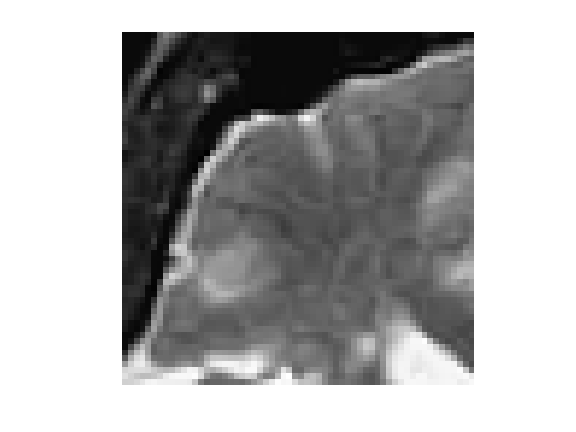Exercise 5

Compute an edge attracting criterion $W(x)>0$, that is small in area of strong gradient.

In :
exo5()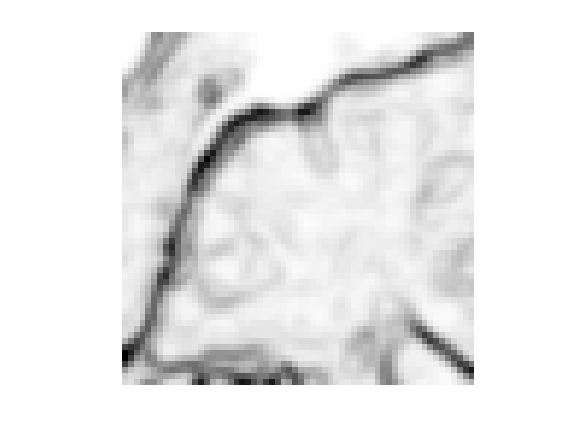In :
%% Insert your code here.


Start and end points $x_0$ and $x_1$.

In :
x0 = 4 + 55i;
x1 = 53 + 4i;


Initial curve $\ga_0$.

In :
p = 128;
t = linspace(0,1,p)';
gamma0 = t*x1 + (1-t)*x0;


Initialize the evolution.

In :
gamma = gamma0;


Display.

In :
clf; hold on;
imageplot(W);
h = plot(imag(gamma([1:end])),real(gamma([1:end])), 'r'); set(h, 'LineWidth', 2);
h = plot(imag(gamma([1 end])),real(gamma([1 end])), 'b.'); set(h, 'MarkerSize', 30);
axis('ij');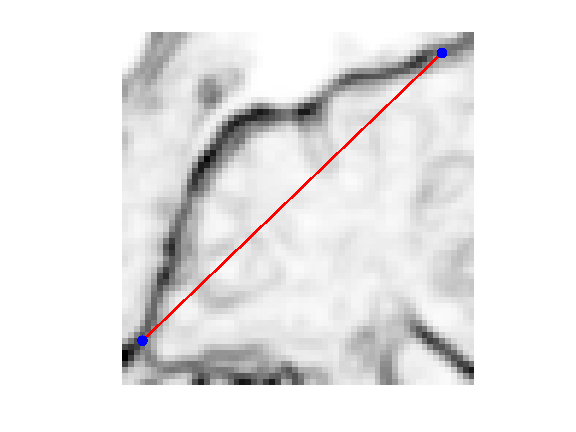Re-sampling for non-periodic curves.

In :
curvabs = @(gamma)[0;cumsum( 1e-5 + abs(gamma(1:end-1)-gamma(2:end)) )];
resample1 = @(gamma,d)interp1(d/d(end),gamma,(0:p-1)'/(p-1), 'linear');
resample = @(gamma)resample1( gamma, curvabs(gamma) );


Time step.

In :
dt = 1/10;


Number of iterations.

In :
Tmax = 2000*4/7;
niter = round(Tmax/dt);


Exercise 6

Perform the curve evolution. Be careful to impose the boundary conditions at each step.

In :
exo6()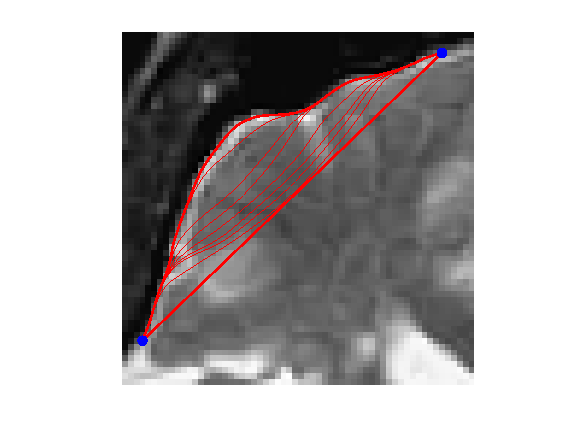In :
%% Insert your code here.